Working with charts
A chart is a graphical representation of data. Charts are particularly useful for summarizing numeric data and showing the relationship between sets of values, called series. For example, a chart can show sales by region, average temperatures by month, or the price of a stock over three months.
Because a chart presents a picture, it reveals trends that are not as apparent in a table. Typically, reports display detail data in a table and summary data in a chart, especially if the detail data spans multiple pages. All charts in Report Studio derive data from the report table.
Report Studio supports animated charts based on HTML5 technology. In previous releases, Report Studio supported static charts and Flash charts, but in the current release, Report Studio supports modifying existing static and Flash charts, if you are using reports that contain this content.
When using a chart, you perform the following tasks:
1 Insert a chart at the table or section header level in a BIRT design.
2 Choose a chart type.
3 Specify the data to present in the chart.
4 Format the chart.
The location in which you insert a chart determines what data the chart displays. For example, in a lengthy report, a chart at the table level can display sales and profit totals for the company, while a chart at the sales office section level can display sales and profit totals for each sales office.
How to insert a chart
To insert a chart in the report, complete the following tasks: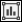To insert a chart at the table level, select the table, then choose
ChartInsert Chart.To insert a chart at a section level, select the section heading, then choose ChartInsert Chart.
When you choose to insert a chart in Report Studio, Chart Builder appears, enabling you to select a chart type, select data for the chart, and format the chart.
Selecting a chart type
Report Studio provides several chart types for use in a BIRT design. After you insert a chart, the first step is to choose the most suitable chart type for the data.The following sections describe the chart types Report Studio supports. Several of the chart types include subtypes.
Working with area charts
An area chart displays data values as a set of points connected by a line, with the area below the line filled. You typically use an area chart to present data that occurs over a continuous period of time. There are three subtypes of area charts: overlay, stacked, and percent stacked.
In an overlay area chart, shown on the left in Figure 6‑1, the areas of multiple series overlap. This chart subtype is not suitable for showing multiple series if the data values overlap. For example in a chart displaying sales values for global territories, if the values in one territory, the U.S., are the highest for every quarter, the data for the U.S. obscures the data for Europe and Asia. Use the overlay area chart if you want to show only one series, for example, only sales for Asia.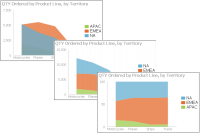Figure 6‑1 Overlay, stacked, and percent stacked area charts
In a stacked area chart, multiple series are stacked vertically, as shown in the chart at the center in Figure 6‑1. The example shows that the stacked area chart is suitable for the data because the chart displays totals for all series as well as the proportion that each series contributes to the total. The height of the top line shows the total value for each quarter. Each shaded area represents the sales amount for a specific region.
In a percent stacked area chart shown on the right in Figure 6‑1, multiple series are stacked vertically, and the values appear as a percentage of the whole. As the chart in Figure 6‑1 shows, the sales values are displayed in percentages instead of the actual numbers, as shown in the previous area charts. The percent stacked area chart is meaningful only when displaying and comparing multiple series.
Working with bar charts
A bar chart, by default, displays data values as a set of vertical bars, but you can transpose the axes to display horizontal bars. A bar chart is useful for displaying data side by side for easy comparison. There are three subtypes of bar charts: side‑by‑side, stacked, and percent stacked. The stacked and percent stacked bar charts are functionally similar to the stacked area chart and percent stacked area chart subtypes.
In a side‑by‑side bar chart, multiple series appear as side‑by‑side bars, as shown in the chart on the left in Figure 6‑2. This bar chart uses the same data as the area charts, shown in the earlier sections.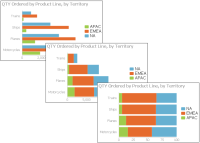Figure 6‑2 Side‑by‑side, stacked, and percent stacked bar charts
In a stacked bar chart, multiple series are stacked vertically, as shown in the chart in the center in Figure 6‑2. The stacked bar chart shows totals for each category, each quarter in this example, as well as the proportion that each series contributes to the total.
In a percent stacked bar chart, multiple series are stacked vertically, and the values are shown as a percentage of the whole. As shown in the chart on the right in Figure 6‑2, the sales values are shown in percentages instead of the actual numbers as shown in the previous bar charts.
Like the percent stacked area chart, the percent stacked bar chart is meaningful only when displaying and comparing multiple series. Do not use this chart subtype if you are displaying only one series, for example, only sales for Asia.
Working with column charts
A column chart displays data values as a set of vertical bars, with categories on the horizontal axis, and values on the vertical axis. This layout is useful for displaying data side by side for easy comparison, as shown in the chart on the left in Figure 6‑3.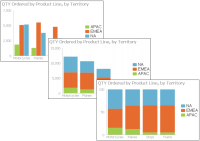Figure 6‑3 Side‑by‑side, stacked, and percent stacked column charts
This chart supports a stacked or percent stacked chart subtype that also shows the relationships of values in each category to the whole, as shown in the charts in the center and on the right in Figure 6‑3.
Working with line charts
A line chart displays data values as a set of points that are connected by a line. You typically use line charts to present large amounts of data that occur over a continuous period of time. A line chart is the most basic type of chart in finance. The chart on the left in Figure 6‑4 shows an example of an overlay line chart.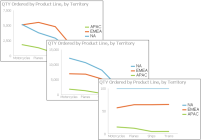Figure 6‑4 Overlay, stacked, and percent stacked line charts
A line chart is similar to an area chart, except that the line chart does not fill in the area below the line. In an overlay line chart, multiple series appear as overlapping lines, as shown in the chart on the left in Figure 6‑4. The line chart supports stacked and percent stacked subtypes.
In a stacked line chart, multiple series are stacked vertically, as shown in the chart in the center in Figure 6‑4. The stacked line chart shows totals for each series, as well as the proportion that each series contributes to the total. In a stacked area chart, the filled‑in areas provide a clear visual cue that each part is compared to the whole.
In a percent stacked line chart, multiple series are stacked vertically and the values are shown as a percentage of the whole. As shown in the chart on the right in Figure 6‑4, the sales values appear in percentages instead of numbers. Like the percent stacked area chart, the percent stacked line chart makes sense only when displaying and comparing multiple series. Do not use this chart subtype if you are displaying only one series, for example, only sales for EMEA. Both the stacked line chart and the percent stacked line chart are not as effective as their area chart counterparts.
In addition, as the example shows, a user can easily misinterpret the data in a stacked line chart. There is no obvious indication that the top line shows the total sales amount for each quarter, and the middle line shows the difference in the sales amount between EMEA and APAC.
Working with meter charts
A meter chart displays a value as a needle pointer on a semicircle, called a dial. As Figure 6‑5 shows, a meter chart resembles a speedometer, with tick marks and numbers showing a range of values. In this example, the meter chart displays pointers that represent two values: profit and revenue totals for each territory. A meter chart supports standard and superimposed subtypes.
The standard meter chart typically creates a dashboard effect, which is visually compelling. A standard meter chart displays multiple values in multiple dials, where each dial displays a single value, as shown in the chart on the left in Figure 6‑5. A superimposed meter chart displays multiple values in a single dial, as shown in the chart on the right in Figure 6‑5.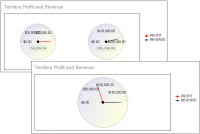Figure 6‑5 A superimposed meter chart showing two values in a single dial
Use the superimposed meter chart type when there are few values to display and when each value is distinct. Duplicate values result in overlapping needles.
Working with pie charts
A pie chart is a circular chart that is divided into sectors or slices. Each sector represents a value that is proportional to the sum of the values. Use a pie chart to show the relationship of parts to the whole, for example, the order quantity each product line contributes to the total, as shown in Figure 6‑6.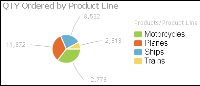Figure 6‑6 A pie chart
Working with doughnut charts
Doughnut charts are similar to pie charts. These are ring charts in the form of segments. Each segment represent a value that is proportional to the total value, as shown in Figure 6‑7.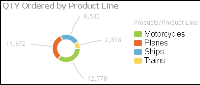Figure 6‑7 A doughnut chart
Working with scatter charts
A scatter chart presents data as xy coordinates by displaying two sets of numeric values as single data points. A scatter chart typically is used to display scientific and statistical data because it shows if there is a relationship between two sets of measurements. For example, use a scatter chart to compare salaries and years of experience or weight and body fat. The more data values you include in a scatter chart, the clearer the trends the data reveals.
The scatter chart in Figure 6‑8 shows the relationship between quantity ordered and profit over three years. Each pair of values, quantity ordered and profit, is plotted as a single xy value.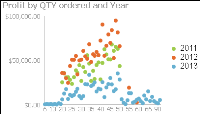Figure 6‑8 A scatter chart
Working with stock charts
A stock chart displays a stock’s open, close, high, and low values for a set of trading dates. A stock chart can show the data for one stock or for multiple stocks. Although a stock chart is typically used to display stock data, you can also use it to chart other values such as four daily temperature values for a set of dates: high, low, sunrise, sunset. The stock chart has two subtypes: the candlestick and bar stick stock charts.
A candlestick stock chart consists of a series of boxes with lines extending up and down from the ends, as shown in the chart on the left in Figure 6‑9. The top and bottom points of each line indicate the high and low values, respectively. The top and bottom of each box indicate the open and close values. If the close value is higher than the open value, the box is white. If the open value is higher than the close value, the box is shaded. This style enables you to see immediately whether a value posted a gain or a loss for a given day.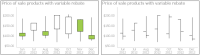Figure 6‑9 Candlestick and bar stick stock charts
A bar stick stock chart consists of a series of vertical bars with horizontal tick marks, as shown in the chart on the right in Figure 6‑9. The top and bottom points of each bar indicate the high and low values, respectively. The horizontal tick marks indicate the open and close values. The tick mark on the left of the bar is the open value. The tick mark on the right of the bar is the close value. A bar stick stock chart typically shows the change in price over a period of time. The candlestick stock chart shows the gain or loss pattern more clearly than the bar stick stock chart.
Radar charts compare the aggregate values of one or more series of data. A separate spoke from the chart center is shown for each category and each spoke is connected by an arc. A line is drawn connecting the data values for each spoke giving the chart a star-like appearance. Radar charts have two subtypes: standard radar charts and spider radar charts, shown in Figure 6‑10.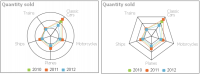Figure 6‑10 Standard and spider radar charts
A spider radar chart connects the outer spokes using lines and a standard radar chart uses arcs, as shown in the chart on the left in Figure 6‑10. Radar charts are most effective for small data sets containing only a few hundred data rows. For larger data sets, or those containing a time series, use a line chart.
Working with difference charts
A difference chart shows a variation between two sets of data by shading the area between points of comparison. A difference chart is useful when you want to display the deviation between two sets of data values, as shown in Figure 6‑11.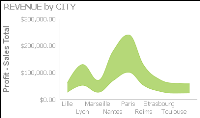Figure 6‑11 A difference chart
Working with Gantt charts
A Gantt chart graphically presents project scheduling information by displaying the duration of tasks. One axis contains the time series, and the other contains tasks. You can use color-coded bars to show the planned duration of the stages to complete the tasks. Bars can use multiple colors to differentiate between stages. Gantt charts use symbols on bars to mark beginning and ending dates. The colors of the bars represent the task status. Optionally, markers designate the start and end dates of tasks. Figure 6‑12 shows a Gantt chart.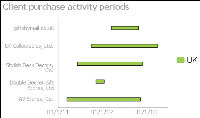Figure 6‑12 A Gantt chart
Working with bubble charts
A bubble chart displays three sets of numeric data values at a time: two values are data points with x-y coordinates on the axes, and the third value defines the size of the bubble at each point. A typical use of a bubble chart is to present financial data, such as quantity sold, profit margin, and total profit sales of multiple product lines. Figure 6‑13 shows a bubble chart.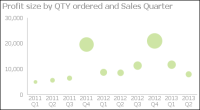Figure 6‑13 A bubble chart